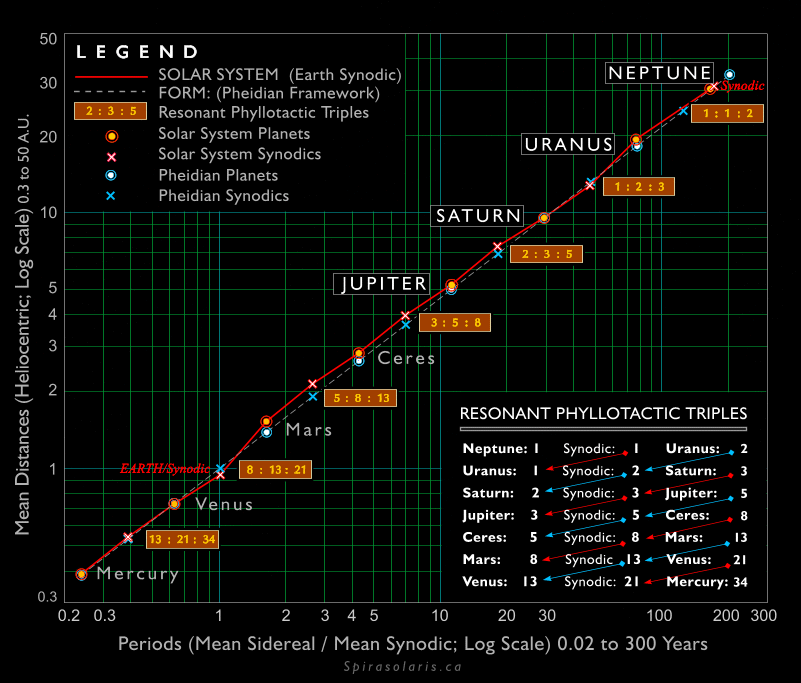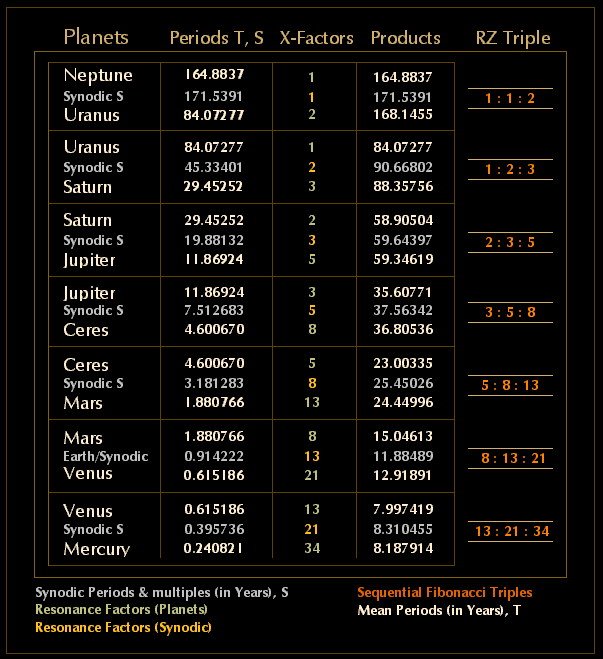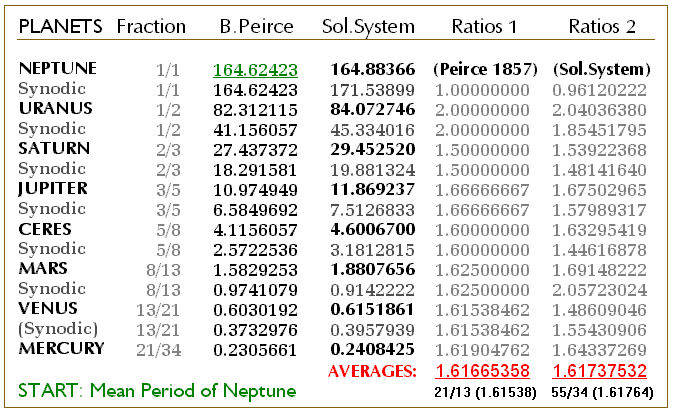Selected Graphics from SPIRASOLARIS: Form and PhyllotaxisFigure 2. Phyllotactic Resonant Triples in the Solar System

Thus in Table 6 below the mean sidereal periods of revolution and intervening mean synodic periods are multiplied by the corresponding X-Factors (numbers of mean sidereal and mean synodic periods) to produce the resonant period for each pair of planets with the latter also providing the corresponding Resonant (RZ) Triples. There is more that could be shown here, including resonant triple variants for Earth and its bracketing synodic periods, and also further investigations involving slightly improved correspondances obtained from the use of aphelion and and perihelion periods rather than mean values.Table 6. Resonant Phyllotactic Triples, Mercury to Neptune (Mean values; Ceres included, Earth Synodic)

Although not identical, the resonant periods obtained from Solar System mean values essentially follow the fibonacci series from its beginning values out to the number 34 (i.e., 1, 1, 2, 3, 5, 8, 13, 21, 34,..) and even further (embracing the next fibonacci number 55) if IMO, the Inter-Mercurial Object from Part II is included. Thus for the four superior planets Neptune, Uranus, Saturn and Jupiter the first fibonacci resonant sequence [ 1 : 1 : 2 ] is followed by [ 1 : 2 : 3 ] then [ 2 : 3 : 5 ] and so on down to the last resonant triple [ 13 : 21 : 34 ] between Venus and Mercury (Figure 2 above).

IV.1.   THE PHYLLOTACTIC DIVISORS AND SYNODIC PERIODS
For purposes of comparison modern values for the mean sidereal periods and the calculated synodic periods for the planets are given in the Sol.System column. A second comparison lists the ratios for each step followed by the average values obtained from each column. As can be seen, the latter are close to fibonacci ratios of 21/13 and 55/34 with resulting pheidian approximations of 1.61665353 and 1.61737532 respectively despite the variance in the individual ratios.Table 3. Benjamin Peirce's Phyllotactic Divisors

PARE
NT PAPER:

SPIRASOLARIS: Form and Phyllotaxis